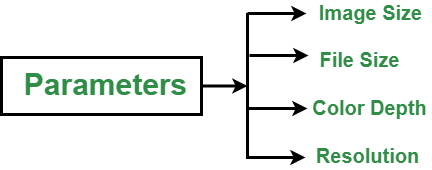Open in App
Not now

# Basic Attributes of an Image

• Last Updated : 26 Aug, 2021

Image :

Most of the multimedia applications have to handle the various types of images. Digital image is the integral part of almost all multimedia applications. The digital image is sampled and mapped as a grid of dots or pictures elements. Each pixel is assigned a tonal value(black, white, shades of gray or color) which is represented by binary code. the binary digits for each pixel are stored in a sequence by a computer. To reduce the storage requirement, the image is often stored in a compressed file format.

Basic Attributes of Image :

The quality of an image depends upon certain parameters. These are as follows:Image Size:

Every image has a width and length expressed either using physical dimensions (cm, inches, etc.) or logical dimensions (pixels). For editing and manipulation of the image, the logical size is considered but if you are going to print it, uses physical dimensions. The dimension of the pixel is the horizontal and vertical measurements of an image that is expressed in pixels. The pixel dimensions can be determined by multiplying both the width and height by the dpi.

`Pixel =  ( height and width ) * dpi`

Example : An 8″ x 10” document that is scanned at 300 dpi has the pixel dimensions of 2,400 pixels by 3,000 pixels.

File Size :

The file size of the image is the digital size of an image file in kilobytes (kb), megabytes (mb), or gigabytes (gb). It is proportional to the pixel dimension of the image. Images with more pixels require more disk space to store.

Factors affecting the file size :

• The larger the size of a file, the more time it will take to load.
• The file format also affects the file size.

The file size is calculated by multiplying the surface area of a document to be scanned by the but depth and the dpi2 .As we know the file size is represented in bytes which is made up of 8 bits, so divide by 8 :

`File Size = (height x width x bit depth x dpi2)/8`

If the pixel dimensions are given, multiply them by each other and the bit depth to determine the number of bits in an image file.

`File Size = (pixel dimensions x bit depth)/8`

Example : 24-bit image is captured with a digital camera with a pixel dimension of 2,048 x 3,072 equals 18,874,368 bytes.

Clock depth/bit depth :

The number of possible shades or tonal degrees that a color can have from black to white.

Example :

• Bitonal image is 1-bit i.e. two colors.
• Grayscale images are typically 8-bit i.e. 256 values.
• Color images are typically 24 bit colors i.e. 3 colors, 8 bit per color and 16 million values.

Bit depth measures how many unique colors are available in an image’s color palette in terms of numbers of 0’s and 1’s. For a grayscale images, the bit depth counts how many shades of grays are available.

Example : In a 2-bit image, there are four possible combinations: 00,01,10,11.

• If “00” represents black.
• If “11” represents white.
• If “01” represents dark gray.
• If “10” represents light gray.

The bit depth is two, but the number of tones that can be represented is 22 i.e. 4.

Most color images from digital cameras have 8-bits per channel. This allows for 256 different combinations. When all the three primary colors are combined at each pixel, this allows for as many as 256*256*256 = 16,777,216 different colors, or “true colors”.

```1 bit = 2 tones
2 bits = 4 tones
3 bits = 8 tones
4 bits= 16 tones
8 bites = 256 tones
16 bites = 65,536 tones
24 bites = 16.7 million tones```

Resolution :

It refers to the number of pixels in an image. A pixel is actually a unit of the digital image. More the count of pixels, the higher the resolution of the image. Higher the resolution of the image, the quality of the image is high. It is also referred to as Pixel Count Resolution. It is expressed in megapixels or pixel dimensions. The term megapixels simply means million of pixels. One megapixel value equals 1 million of pixels. Spatial resolution refers to the number of independent pixel values per un it length. The spatial resolution of an image is commonly referred to in terms of dpi.

My Personal Notes arrow_drop_up